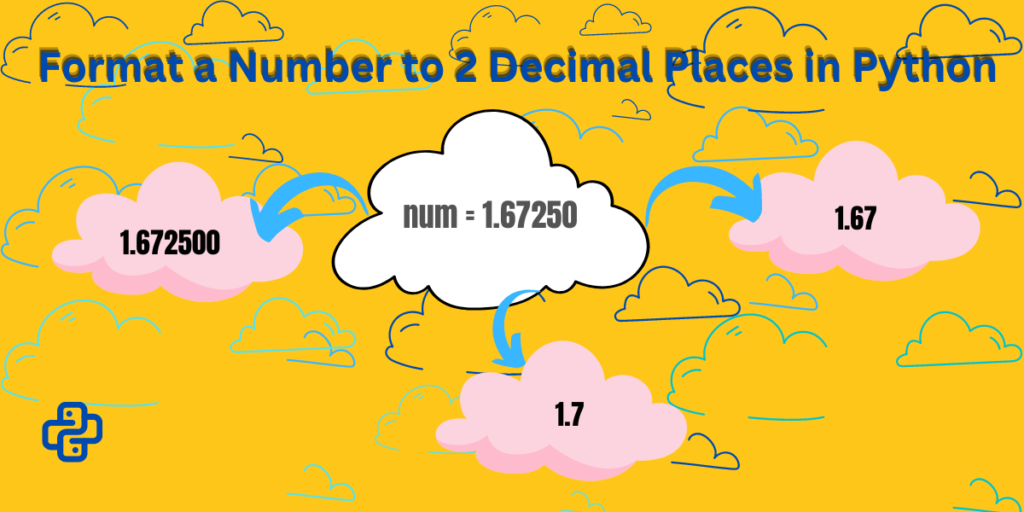# How to Format a Number to 2 Decimal Places in Python?Decimal Places are in the float type datatype. Python Number datatypes store the numeric value. There are three numeric types in Python: `int`, `float`, `complex`. They are immutable. The `int` integer type is the number zero, a positive natural number, or a negative integer. A `float` type is a number, positive or negative, containing decimal places. A `complex` number is written with an imaginary part, and the imaginary part is “j”.

## Data Types in Python

Python has various built-in data types to store different types of data. Let’s take a look at them.

### Get the Datatype

Python provides the `type()` function to know the datatype of the object. The `type()` function returns the object type if only one object parameter is passed and a new kind of 3 parameters is passed.

#### Syntax

```type(object)

type(name, bases, dict)
```

Let’s take a look at an example for general understanding.

```x = 5
print(type(x))

y=7.0
print(type(y))

s = 'abc'
print(type(s))

dict = {"name": "Dhushi"}
print(type(dict))

l= ['a',1,5.3]
print(type(l))
```

Here we are assigned different data types to the variables to check which value belongs to which data type.

1. assigned numeric value 5 to variable x
2. decimal value 7.0 to y variable
3. the character string “abc” to s variable
4. key-value pair inside curly braces to variable dict
5. lastly, values enclosed in square brackets `[]` to variable l

#### Output:

In the output, we can see the different datatypes of values assigned to different variables.

1. output for x=5 is `int`. Python has no restriction on the length of an integer it can be 8, 10, 999, -5786, etc.
2. 7.0 is a float-type value as it has a decimal in it.
3. “abc” is string type as it contains the characters within the double quotes(it can be single quotes also)
4. A dictionary holds a key: value pair, so the type is dict for variable dict, which means it is a dictionary
5. lastly, Lists are used to store multiple items in a single variable, it is written using square brackets` []`. So, the type for the l variable is the list.

Let’s move on to our topic of how we can format a number to 2 decimal places.

Get to know more about Datatypes in Python.

## Format a Number to 2 Decimal Places

When working with float values containing a decimal, we sometimes want to have only one or two digits or digits as per requirements after the decimal point. So the question is how to do that? It is pretty simple by using %f formatter or `str.format()` with “{:.2f}” as a string and float as a number.

Let’s take a look at the first method.

### Using %f formatter

The `%f` formatter is used to input float values or numbers with values after the decimal place. It allows us to tell how many digits we require after decimal and round up the value.

#### Example 1: printing the value of the variable `num` as a decimal number with six decimal places

```num = 25.21997

print("%f" % num)
```

assigned a float value to variable num, and while printing the output the `%f` formatter is written inside quotation marks, and the % modulo operator separates it from the float number `"%f" % num`.

In the output, we get one extra zero at the end, and the output contains the full float value as we have not provided how many digits we want after the decimal.

#### Example 2: how to get the value of digits after the decimal

In this example, we will look at how to get the value of digits after the decimal.

```num = 1.672500

print("%.1f" % num)
print("%.2f" % num)
```

Assigned the float value to the variable num. Then we print output for a value containing 1 digit and 2 digits after the decimal. Like before when we only used %f for the full float value to be showcased, but here we are adding “%.1f” for the 1 digit to appear after the decimal and “%.2f” for the 2 digits to appear after the decimal.

In the result, we can see how “%.1f” produced result 1.7, it has rounded up the value. In the second case, we get 1.67 from “%.2f“.

### Using str.format()

Here we are going to see how to present 2 places value after a decimal using `str.format()`.

```num = 7.123456
print("%1.2f " % num)
print("{:.2f}".format( num ))
```
1. In this piece of code, assign a float value to variable num.
2. then we print the value from the style using %f formatter.
3. lastly, `str.format() `has been used to give the 2 decimal place value. Here “{:.2f}” is a string where we have mentioned .2f, which means 2 digits after the decimal in the float number. Then we provide our float value on which the action will take place in the format function.

In the result, we are getting the same result using two different ways.

You can check The Python float() Method for more knowledge on the float method.

## Conclusion

In this article, we learn how to format a number to 2-decimal places by using two ways. You can show the result using %f formatted, written inside quotation marks, and the % modulo operator separates it from the float number “%f” % num. Also, using str.format() is simple, where you have to write within the curly braces how many places you want after the decimal followed by the variable name in the format function.

Here’s the official Python documentation to help you understand decimal in Python.